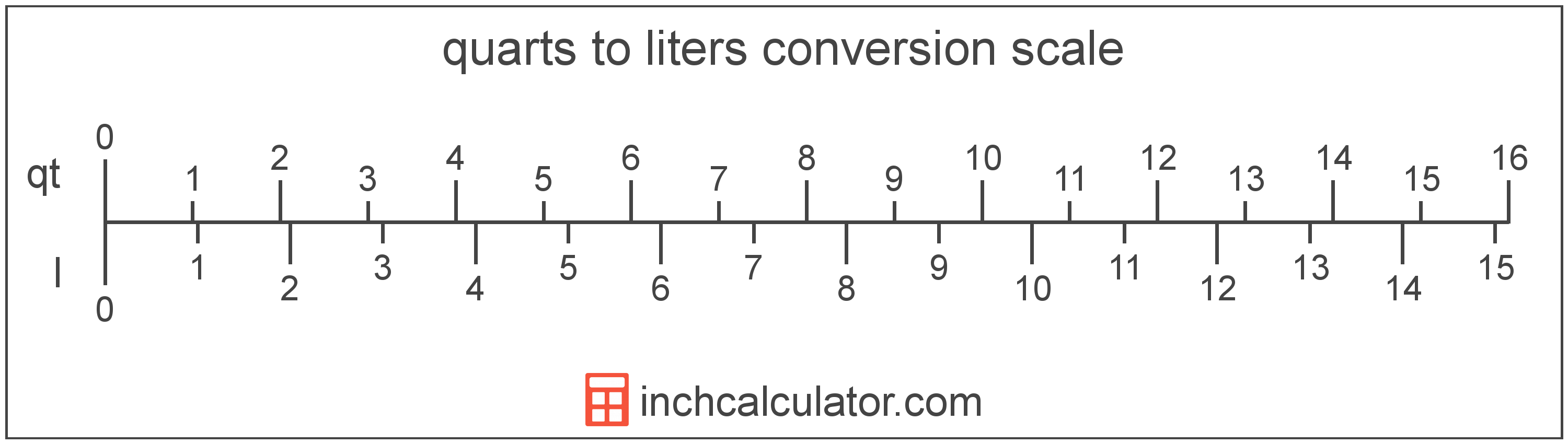# Liters to Quarts Converter

Enter the volume in liters below to get the value converted to quarts.

Results in Quarts:1 L = 1.056688 qt

Do you want to convert quarts to liters?

## How to Convert Liters to Quarts

To convert a measurement in liters to a measurement in quarts, multiply the volume by the following conversion ratio: 1.056688 quarts/liter.

Since one liter is equal to 1.056688 quarts, you can use this simple formula to convert:

quarts = liters × 1.056688

The volume in quarts is equal to the volume in liters multiplied by 1.056688.

For example, here's how to convert 5 liters to quarts using the formula above.
quarts = (5 L × 1.056688) = 5.283441 qt### How Many Quarts Are in a Liter?

There are 1.056688 quarts in a liter, which is why we use this value in the formula above.

1 L = 1.056688 qt

## What Is a Liter?

A liter is a unit of volume equal to 1,000 cubic centimeters or 0.264172 US gallons. The liter is a special name defined for the cubic decimeter and is exactly equal to the volume of one cubic decimeter (1 decimeter is 1/10 of a meter, or 10 centimeters).

The liter is an SI accepted unit for volume for use with the metric system. A liter is sometimes also referred to as a litre. Liters can be abbreviated as L, and are also sometimes abbreviated as l or . For example, 1 liter can be written as 1 L, 1 l, or 1 ℓ.

## What Is a Quart?

The US liquid quart is a unit of fluid volume equal to one fourth of a gallon, two pints, or four cups.

The liquid quart should not be confused with the dry quart (US) or the imperial quart, which are different units. However, when someone refers to a volume in quarts, they typically mean the US liquid quart.

The quart is a US customary unit of volume. Quarts can be abbreviated as qt; for example, 1 quart can be written as 1 qt.

## Liter to Quart Conversion Table

Table showing various liter measurements converted to quarts.
Liters Quarts
1 L 1.0567 qt
2 L 2.1134 qt
3 L 3.1701 qt
4 L 4.2268 qt
5 L 5.2834 qt
6 L 6.3401 qt
7 L 7.3968 qt
8 L 8.4535 qt
9 L 9.5102 qt
10 L 10.57 qt
11 L 11.62 qt
12 L 12.68 qt
13 L 13.74 qt
14 L 14.79 qt
15 L 15.85 qt
16 L 16.91 qt
17 L 17.96 qt
18 L 19.02 qt
19 L 20.08 qt
20 L 21.13 qt
21 L 22.19 qt
22 L 23.25 qt
23 L 24.3 qt
24 L 25.36 qt
25 L 26.42 qt
26 L 27.47 qt
27 L 28.53 qt
28 L 29.59 qt
29 L 30.64 qt
30 L 31.7 qt
31 L 32.76 qt
32 L 33.81 qt
33 L 34.87 qt
34 L 35.93 qt
35 L 36.98 qt
36 L 38.04 qt
37 L 39.1 qt
38 L 40.15 qt
39 L 41.21 qt
40 L 42.27 qt

## References

1. National Institute of Standards and Technology, Units outside the SI, https://physics.nist.gov/cuu/Units/outside.html